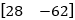Multiplying Two Matrices

Matrix Multiplication

Multiplying Two Matrices

To find the product of two matrices, such as matrix M and N, we need to look at the first row for matrix M and the first column for matrix N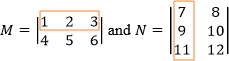Once you find them, multiply corresponding elements, and find the sum of their products.

(17) + (29) + (311) = 59

This is the first element of the resultant matrix.

Now locate the first row for matrix M and the second column for matrix N. How can you find the second element for the resultant matrix?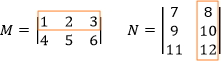Once you find them, multiply corresponding elements, and find the sum of their products.

(18) + (210) + (312) = 64

This is the second element of the resultant matrix.

For the next step we are going to repeat the same procedure, but this time we will work with the second row of M and the first column of N, followed by locating the second row of M and second column of N, and we obtain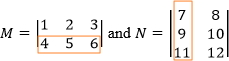(47) + (59) + (611) = 132

This is the third element of the resultant matrix.

and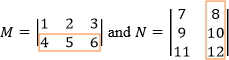(48) + (510) + (612) = 154

This is the fourth element of the resultant matrix.

Therefore, the resultant matrix is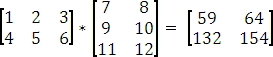Can These Matrices Be Multiplied Together?

If M is an xy matrix,and N is a yz matrix, the product of M * N is an xz matrix. To determine whether or not matrix M and N can be multiplied we need to look at the size of each matrix. Since M is an xy and N is a yz matrices, y needs to be in the same in order to be a multipliable matrix. Also x and z will determine the size of the resultant. Therefore

M  *  N  =  MN
xy  *  yz  =  xz

Equal Order of MN

So, if matrix M is 34 and matrix N is a 42, then M * N is possible since 4 = 4, and the size of the resultant matrix is 32.

N * M is not possible since 2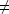3.

It is important to know that if M * N is possible, it does not mean that N * M is possible as well.

Practice

Multiply if possible.

1)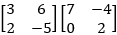2)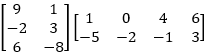3)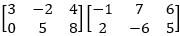4)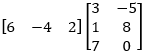1)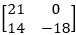2)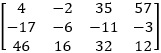3)   Not possible

4)